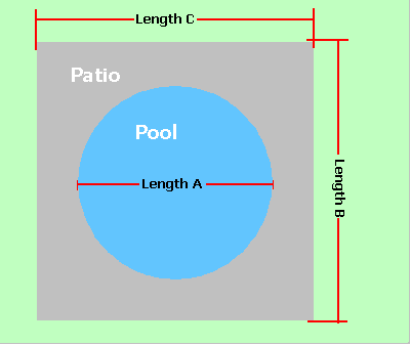Spike's Calculators

# Square Rectangular Shaped Concrete Patio - Round Pool - Imperial Measurements

Calculate the amount of concrete needed to pour a slab around a round pool using imperial measurements. The pool patio has a rectangular/square shape.

Includes a result in square feet of the patio area and the outer perimeter of this area in lineal feet. The square footage of this patio area can be used to estimate for different materials needed and the outer perimeter to estimate the fencing material.### Square/Rectangular Slab - Round Pool

Diameter of Pool Length A ft
Length of Outer Area Length B ft
Length of Outer Area Length C ft
Required Slab Depth in

#### Results:

 Area of Pool ft² Area of Pool Patio ft² Outer Perimeter lin ft Concrete Needed in Cubic Yards yd³ Concrete Needed in Cubic Metres m³

#### Calculations

1. the diameter of the pool (length A in feet)
2. the length of the overall area (length B in feet)
3. the width of the overall area (length C in feet)
4. depth of concrete slab (inches)

#### Results

1. square footage of the pool area
2. square footage of the patio area around the pool
3. outer perimeter of the patio area (lineal feet)
4. concrete needed in cubic yards
5. concrete needed in cubic metres